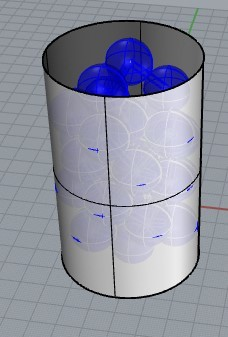# How to make connection between sphere and wall at certain distance with cylinder

Hi guys,

This question is actually related and a continuation to my previous question, which is given in the following link:

This time I want to make not only particle-to-particle connection (which was done previously and included in this case as well) but I add a wall around the bunch of spheres as the following figure and connect certain spheres and the wall if their distances meet the criterion. The cylinder wall dimension is set at d = 31.5 and l = 100. Just as the previous case, the connecting geometry is a cylinder whose radius is 0.08 times the sphere`s radius.The criterion is also similar to the previous case, which is given as follows.

gap = rs.Distance(point1, wall) - radius1;
tolerance = 0.050
if val <= tolerance, the connection shall be formed

I made a script for this case, but as seen I have yet added codes for wall-sphere cylindrical connector because I don`t know codes needed to make one. Any help would be appreciated.

``````import rhinoscriptsyntax as rs
import math as math

def isclose(a, b, rel_tol=1e-09, abs_tol=0.0):
return abs(a-b) <= max(rel_tol * max(abs(a), abs(b)), abs_tol)

# determination of particle-particle bridge is given as follows
tolerance = 0.050
return (isclose(val, tolerance) or (val < tolerance))

#sphere coordinates

points = []
points.append([3.8004, -10.7703, -14.7366])
points.append([6.89814, 9.90892, -14.7361])
points.append([-14.1991, -1.3038, -14.7362])
points.append([-2.50297, -1.28871, 1.70578])
points.append([12.3037, -17.4367, 2.09313])
points.append([21.1603, -2.79959, -8.81348])
points.append([-11.5506, -18.1149, -4.23054])
points.append([-11.1571, 18.5256, -14.736])
points.append([4.63671, 21.0576, 1.71328])
points.append([-19.803, 8.74576, 1.62201])
points.append([19.4772, 8.88409, 7.33124])
points.append([19.7547, -8.05903, 18.1097])
points.append([8.40554, -19.6233, 29.834])
points.append([19.879, 7.92851, 30.1251])
points.append([4.80376, 21.0154, 28.916])
points.append([-14.0158, -14.7737, 29.5774])
points.append([-19.7362, -8.70016, 11.4009])
points.append([-3.47392, -21.1316, 13.8154])
points.append([-20.8642, 5.75207, 25.1795])
points.append([3.10321, 2.98932, 20.421])
points.append([-9.83524, 19.2703, 15.4012])
points.append([-21.4772, -2.41992, 43.4234])
points.append([19.6639, -8.27222, 41.8506])
points.append([-12.3412, 17.7824, 38.6932])
points.append([-2.90253, 21.4069, 55.9492])
points.append([-0.923667, 0.666779, 39.873])
points.append([-3.82574, -21.0765, 45.5909])
points.append([14.4364, 15.8948, 47.644])
points.append([-7.73744, -5.57666, 57.6097])

for i in range(len(points)):

#forming inter-particle connections
#don`t forget to add cylinder direction vector to connect each center point

for i in range(len(points)):
for j in range(i+1, len(points)):
direction_vector = rs.VectorCreate(points[j], points[i])
cylinder_plane = rs.PlaneFromNormal(points[i],direction_vector)

#cylinder wall
cylinder = rs.AddCylinder([0,0,-30], 100, 31.5, False)

if rs.IsCylinder(cylinder):

# determination of particle-wall bridge is given as follows